# Existence and uniqueness of positive solution to singular fractional differential equations

## Abstract

In this paper, we discuss the existence and uniqueness of a positive solution to the following singular fractional differential equation with nonlocal boundary value conditions:

$\left\{\begin{array}{c}{D}_{0+}^{\alpha }u\left(t\right)+f\left(t,u\left(t\right)\right)=0,\phantom{\rule{1em}{0ex}}0

where $1<\alpha \le 2$, $0<\beta <\alpha -1$, $0<{\xi }_{1}<\cdots <{\xi }_{m-2}<1$ with ${\sum }_{i=1}^{m-2}{\eta }_{i}{\xi }_{i}^{\alpha -\beta -1}<1$, ${D}_{0+}^{\alpha }$ is the standard Riemann-Liouville derivative, f may be singular at $t=0$, $t=1$, and $u=0$.

MSC:34B10, 34B15.

## 1 Introduction

In this paper, we consider the following fractional differential equation:

$\left\{\begin{array}{c}{D}_{0+}^{\alpha }u\left(t\right)+f\left(t,u\left(t\right)\right)=0,\phantom{\rule{1em}{0ex}}0
(1.1)

where $1<\alpha \le 2$, $0<\beta <\alpha -1$, $0<{\xi }_{1}<\cdots <{\xi }_{m-2}<1$ with ${\sum }_{i=1}^{m-2}{\eta }_{i}{\xi }_{i}^{\alpha -\beta -1}<1$, ${D}_{0+}^{\alpha }$ is the standard Riemann-Liouville derivative, $f\in C\left(\left(0,1\right)×\left(0,+\mathrm{\infty }\right)\to \left[0,+\mathrm{\infty }\right)\right)$ may be singular at $t=0$, $t=1$, and $u=0$. In this paper, by a positive solution to (1.1), we mean a function $u\in C\left[0,1\right]$ which satisfies ${D}_{0+}^{\alpha }u\in L\left(0,1\right)$, positive on $\left(0,1\right]$ and satisfies (1.1).

Recently, many results were obtained dealing with the existence of solutions for nonlinear fractional differential equations by using the techniques of nonlinear analysis; see  and references therein. The multi-point boundary value problems (BVP for short) have provoked a great deal of attention, for example . In , the authors discussed some positive properties of the Green function for Direchlet-type BVP of nonlinear fractional differential equation

$\left\{\begin{array}{c}{D}_{0+}^{\alpha }u\left(t\right)+f\left(t,u\left(t\right)\right)=0,\phantom{\rule{1em}{0ex}}0
(1.2)

where $1<\alpha <2$, ${D}_{0+}^{\alpha }$ is the standard Riemann-Liouville derivative, $f\in C\left(\left[0,1\right]×\left[0,+\mathrm{\infty }\right)\to \left[0,+\mathrm{\infty }\right)\right)$. By using the Krasnosel’skii fixed point theorem, the existence of positive solutions were obtained under suitable conditions on f.

In , the authors investigated the existence and multiplicity of positive solutions by using some fixed point theorems for the fractional differential equation

$\left\{\begin{array}{c}{D}_{0+}^{\alpha }u\left(t\right)+f\left(t,u\left(t\right)\right)=0,\phantom{\rule{1em}{0ex}}0
(1.3)

where $1<\alpha \le 2$, $0\le \beta \le 1$, $0<\xi <1$, $0\le a\le 1$ with $a{\xi }^{\alpha -\beta -2}<1-\beta$, $0\le \alpha -\beta -1$, $f:\left[0,1\right]×\left[0,+\mathrm{\infty }\right)\to \left[0,+\mathrm{\infty }\right)$ satisfied Carathéodory type conditions.

In [20, 21], the authors considered the fractional differential equation given by

$\left\{\begin{array}{c}{D}_{0+}^{\alpha }u\left(t\right)+f\left(t,u,{u}^{\prime },\dots ,{u}^{\left(n-2\right)}\right)=0,\phantom{\rule{1em}{0ex}}0
(1.4)

In order to obtain the existence of positive solutions of (1.4), they considered the following fractional differential equation:

$\left\{\begin{array}{c}{D}_{0+}^{\alpha -n+2}v\left(t\right)+f\left(t,{I}_{0+}^{n-2}v\left(t\right),{I}_{0+}^{n-3}v\left(t\right),\dots ,{I}_{0+}^{1}v\left(t\right),v\left(t\right)\right)=0,\phantom{\rule{1em}{0ex}}0
(1.5)

In , $f=q\left(t\right)\left(g+h\right)$, and g, h have different monotone properties. By using the fixed point theorem for the mixed monotone operator, Zhang obtained (1.4) and had a unique positive solution $u\left(t\right)={I}_{0+}^{n-2}v\left(t\right)$ with $v\in Q=:\left\{x\left(t\right):\frac{1}{M}{t}^{\alpha -n+1}\le x\left(t\right)\le M{t}^{\alpha -n+1}\right\}$. But the results are not true since $v\left(t\right)$ is a positive solution of (1.5), and $v\left(1\right)=0$. What causes it lies in the unsuitable using of properties of the Green function.

In , $f\in C\left(\left[0,1\right]×\left[0,+\mathrm{\infty }\right)×{R}^{n-2}\to \left[0,+\mathrm{\infty }\right)\right)$, $f\left(t,{y}_{1},{y}_{2},\dots ,{y}_{n-1}\right)$ is increasing for ${y}_{i}\ge 0$, $i=1,2,\dots ,n-1$. By using the positive properties of the Green function obtained in $\left[10\right]$ and fixed point theory for the ${u}_{0}$ concave operator, the authors obtained the uniqueness of a positive solution for the BVP (1.4).

Motivated by the works mentioned above, in this paper we aim to establish the existence and uniqueness of a positive solution to the BVP (1.1). Our work presented in this paper has the following features. Firstly, the BVP (1.1) possesses singularity, that is, f may be singular at $t=0$, $t=1$, and $u=0$. Secondly, we impose weaker positivity conditions on the nonlocal boundary term, that is, some of the coefficients ${\eta }_{i}$ can be negative. Thirdly, the unique positive solution can be approximated by an iterative scheme.

The rest of the paper is organized as follows. In Section 2, we present some preliminaries and lemmas that will be used to prove our main results. We also develop some new positive properties of the Green function. In Section 3, we discuss the existence and uniqueness of a positive solution of the BVP (1.1), we also give an example to demonstrate the application of our theoretical results.

## 2 Preliminaries

For the convenience of the reader, we present here the necessary definitions from fractional calculus theory. These definitions can be found in recent literature.

Definition 2.1 The fractional integral of order $\alpha >0$ of a function $u:\left(0,+\mathrm{\infty }\right)\to R$ is given by

${I}_{0+}^{\alpha }u\left(t\right)=\frac{1}{\mathrm{\Gamma }\left(\alpha \right)}{\int }_{0}^{t}{\left(t-s\right)}^{\alpha -1}u\left(s\right)\phantom{\rule{0.2em}{0ex}}ds$

provided the right-hand side is defined pointwise on $\left(0,+\mathrm{\infty }\right)$.

Definition 2.2 The fractional derivative of order $\alpha >0$ of a continuous function $u:\left(0,+\mathrm{\infty }\right)\to R$ is given by

${D}_{0+}^{\alpha }u\left(t\right)=\frac{1}{\mathrm{\Gamma }\left(n-\alpha \right)}{\left(\frac{d}{dt}\right)}^{n}{\int }_{0}^{t}{\left(t-s\right)}^{n-\alpha -1}u\left(s\right)\phantom{\rule{0.2em}{0ex}}ds,$

where $n=\left[\alpha \right]+1$, $\left[\alpha \right]$ denotes the integral part of the number α, provided the right-hand side is pointwisely defined on $\left(0,+\mathrm{\infty }\right)$.

Definition 2.3 By $u\in L\left(0,1\right)$, we mean ${\int }_{0}^{1}|u\left(t\right)|\phantom{\rule{0.2em}{0ex}}dt<\mathrm{\infty }$.

Lemma 2.1 ()

Let $\alpha >0$. Then the following equality holds for $u\in L\left(0,1\right)$, ${D}_{0+}^{\alpha }u\in L\left(0,1\right)$,

${I}_{0+}^{\alpha }{D}_{0+}^{\alpha }u\left(t\right)=u\left(t\right)+{c}_{1}{t}^{\alpha -1}+{c}_{2}{t}^{\alpha -2}+\cdot \cdot \cdot +{c}_{n}{t}^{\alpha -n},$

where ${c}_{i}\in R$, $i=1,2,\dots ,n$, $n-1<\alpha \le n$.

Set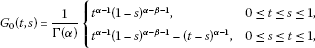(2.1)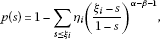(2.2)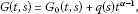(2.3)

where

$q\left(s\right)=\frac{p\left(s\right)-p\left(0\right)}{\mathrm{\Gamma }\left(\alpha \right)p\left(0\right)}{\left(1-s\right)}^{\alpha -\beta -1},\phantom{\rule{2em}{0ex}}p\left(0\right)=1-\sum _{i=1}^{m-2}{\eta }_{i}{\xi }_{i}^{\alpha -\beta -1}.$
(2.4)

For the convenience in presentation, we here list the assumption to be used throughout the paper.

(${H}_{1}$) $p\left(0\right)>0$, $q\left(s\right)\ge 0$ on $\left[0,1\right]$.

Remark 2.1 If ${\eta }_{i}=0$ ($i=1,\dots ,m-2$), we have $p\left(0\right)=1$ and $q\left(s\right)\equiv 0$. If ${\eta }_{i}\ge 0$ ($i=1,\dots ,m-2$) and ${\sum }_{i=1}^{m-2}{\eta }_{i}{\xi }_{i}^{\alpha -\beta -1}<1$, we have $p\left(0\right)>0$ and $q\left(s\right)\ge 0$ on $\left[0,1\right]$.

Lemma 2.2 ()

Assume that $g\in L\left(0,1\right)$ and $\alpha >1\ge \beta \ge 0$. Then

${D}_{0+}^{\beta }{\int }_{0}^{t}{\left(t-s\right)}^{\alpha -1}g\left(s\right)\phantom{\rule{0.2em}{0ex}}ds=\frac{\mathrm{\Gamma }\left(\alpha \right)}{\mathrm{\Gamma }\left(\alpha -\beta \right)}{\int }_{0}^{t}{\left(t-s\right)}^{\alpha -\beta -1}g\left(s\right)\phantom{\rule{0.2em}{0ex}}ds.$

Lemma 2.3 Assume (${H}_{1}$) holds, and $y\in L\left(0,1\right)$. Then the unique solution of the problem

$\left\{\begin{array}{c}{D}_{0+}^{\alpha }u\left(t\right)+y\left(t\right)=0,\phantom{\rule{1em}{0ex}}0
(2.5)

is

$u\left(t\right)={\int }_{0}^{1}G\left(t,s\right)y\left(s\right)\phantom{\rule{0.2em}{0ex}}ds,$

where $G\left(t,s\right)$ is called the Green function of BVP (2.5).

Proof From Lemma 2.1, we have the solution of (2.5) given by

$u\left(t\right)=-{I}_{0+}^{\alpha }y\left(t\right)+{c}_{1}{t}^{\alpha -1}+{c}_{2}{t}^{\alpha -2}.$

Consequently,

$u\left(t\right)=-\frac{1}{\mathrm{\Gamma }\left(\alpha \right)}{\int }_{0}^{t}{\left(t-s\right)}^{\alpha -1}y\left(s\right)\phantom{\rule{0.2em}{0ex}}ds+{c}_{1}{t}^{\alpha -1}+{c}_{2}{t}^{\alpha -2}.$

From $u\left(0\right)=0$, we have ${c}_{2}=0$.

By Lemma 2.2, we have

${D}_{0+}^{\beta }u\left(t\right)=-\frac{1}{\mathrm{\Gamma }\left(\alpha -\beta \right)}{\int }_{0}^{t}{\left(t-s\right)}^{\alpha -\beta -1}y\left(s\right)\phantom{\rule{0.2em}{0ex}}ds+\frac{{c}_{1}\mathrm{\Gamma }\left(\alpha \right)}{\mathrm{\Gamma }\left(\alpha -\beta \right)}{t}^{\alpha -\beta -1}.$

Therefore,

${D}_{0+}^{\beta }u\left(1\right)=-\frac{1}{\mathrm{\Gamma }\left(\alpha -\beta \right)}{\int }_{0}^{1}{\left(1-s\right)}^{\alpha -\beta -1}y\left(s\right)\phantom{\rule{0.2em}{0ex}}ds+\frac{{c}_{1}\mathrm{\Gamma }\left(\alpha \right)}{\mathrm{\Gamma }\left(\alpha -\beta \right)},$

and

${D}_{0+}^{\beta }u\left({\xi }_{i}\right)=-\frac{1}{\mathrm{\Gamma }\left(\alpha -\beta \right)}{\int }_{0}^{{\xi }_{i}}{\left({\xi }_{i}-s\right)}^{\alpha -\beta -1}y\left(s\right)\phantom{\rule{0.2em}{0ex}}ds+\frac{{c}_{1}\mathrm{\Gamma }\left(\alpha \right)}{\mathrm{\Gamma }\left(\alpha -\beta \right)}{\xi }_{i}^{\alpha -\beta -1}.$

By ${D}_{0+}^{\beta }u\left(1\right)={\sum }_{i=1}^{m-2}{\eta }_{i}{D}_{0+}^{\beta }u\left({\xi }_{i}\right)$, we have

${c}_{1}=\frac{{\int }_{0}^{1}{\left(1-s\right)}^{\alpha -1}y\left(s\right)\phantom{\rule{0.2em}{0ex}}ds-{\sum }_{i=1}^{m-2}{\eta }_{i}{\int }_{0}^{{\xi }_{i}}{\left({\xi }_{i}-s\right)}^{\alpha -\beta -1}y\left(s\right)\phantom{\rule{0.2em}{0ex}}ds}{\mathrm{\Gamma }\left(\alpha \right)p\left(0\right)}=\frac{{\int }_{0}^{1}{\left(1-s\right)}^{\alpha -\beta -1}p\left(s\right)y\left(s\right)\phantom{\rule{0.2em}{0ex}}ds}{\mathrm{\Gamma }\left(\alpha \right)p\left(0\right)}.$

Therefore, the solution of (2.5) is

$u\left(t\right)={c}_{1}{t}^{\alpha -1}-\frac{1}{\mathrm{\Gamma }\left(\alpha \right)}{\int }_{0}^{t}{\left(t-s\right)}^{\alpha -1}y\left(s\right)\phantom{\rule{0.2em}{0ex}}ds={\int }_{0}^{1}G\left(t,s\right)y\left(s\right)\phantom{\rule{0.2em}{0ex}}ds.$

□

Lemma 2.4 The function ${G}_{0}\left(t,s\right)$ has the following properties:

1. (1)

${G}_{0}\left(t,s\right)>0$, for $t,s\in \left(0,1\right)$;

2. (2)

$\mathrm{\Gamma }\left(\alpha \right){G}_{0}\left(t,s\right)\le {t}^{\alpha -1}$, for $t,s\in \left[0,1\right]$;

3. (3)

$\beta {t}^{\alpha -1}h\left(s\right)\le \mathrm{\Gamma }\left(\alpha \right){G}_{0}\left(t,s\right)\le h\left(s\right){t}^{\alpha -2}$, for $t,s\in \left(0,1\right)$, where

$h\left(s\right)=s{\left(1-s\right)}^{\alpha -\beta -1}.$
(2.6)

Proof It is obvious that (1), (2) hold. In the following, we will prove (3).

1. (i)

When $0, noticing $0<\beta <\alpha -1\le 1$, we have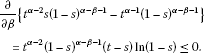(2.7)

Therefore,

$\begin{array}{c}{t}^{\alpha -2}s{\left(1-s\right)}^{\alpha -\beta -1}-\left({t}^{\alpha -1}{\left(1-s\right)}^{\alpha -\beta -1}-{\left(t-s\right)}^{\alpha -1}\right)\hfill \\ \phantom{\rule{1em}{0ex}}\ge {t}^{\alpha -2}s-{t}^{\alpha -1}+{\left(t-s\right)}^{\alpha -1}\hfill \\ \phantom{\rule{1em}{0ex}}=-{t}^{\alpha -2}\left(t-s\right)+{\left(t-s\right)}^{\alpha -1}\ge 0,\hfill \end{array}$

which implies

$\mathrm{\Gamma }\left(\alpha \right){G}_{0}\left(t,s\right)\le h\left(s\right){t}^{\alpha -2}.$
(2.8)

On the other hand, we have

$\frac{\partial }{\partial s}\left\{\beta s+{\left(1-s\right)}^{\beta }\right\}\le 0,\phantom{\rule{1em}{0ex}}s\in \left[0,1\right).$

Therefore, $\beta s+{\left(1-s\right)}^{\beta }\le 1$, which implies

$\left[1-{\left(1-s\right)}^{\beta }\right]\ge \beta s.$

Then

$\begin{array}{rcl}\mathrm{\Gamma }\left(\alpha \right){G}_{0}\left(t,s\right)& =& {t}^{\alpha -1}{\left(1-s\right)}^{\alpha -\beta -1}-{\left(t-s\right)}^{\alpha -1}\\ \ge & {t}^{\alpha -1}{\left(1-s\right)}^{\alpha -\beta -1}-{\left(t-s\right)}^{\beta }{\left(t-ts\right)}^{\alpha -\beta -1}\\ =& \left[1-{\left(1-\frac{s}{t}\right)}^{\beta }\right]{t}^{\alpha -1}{\left(1-s\right)}^{\alpha -\beta -1}\\ \ge & \left[1-{\left(1-s\right)}^{\beta }\right]{t}^{\alpha -1}{\left(1-s\right)}^{\alpha -\beta -1}\ge \beta {t}^{\alpha -1}h\left(s\right).\end{array}$
(2.9)
1. (ii)

When $0, we have

$\begin{array}{rcl}\mathrm{\Gamma }\left(\alpha \right){G}_{0}\left(t,s\right)& =& {t}^{\alpha -1}{\left(1-s\right)}^{\alpha -\beta -1}={t}^{\alpha -2}t{\left(1-s\right)}^{\alpha -\beta -1}\\ \le & {t}^{\alpha -2}s{\left(1-s\right)}^{\alpha -\beta -1}=h\left(s\right){t}^{\alpha -2}.\end{array}$
(2.10)

On the other hand, clearly we have

$\mathrm{\Gamma }\left(\alpha \right){G}_{0}\left(t,s\right)={t}^{\alpha -1}{\left(1-s\right)}^{\alpha -\beta -1}\ge \beta {t}^{\alpha -1}h\left(s\right).$
(2.11)

(2.8)-(2.11) implies (3) holds. □

By Lemma 2.4 we have the following results.

Lemma 2.5 Assume (${H}_{1}$) holds, then the Green function defined by (2.3) satisfies:

1. (1)

$G\left(t,s\right)>0$, $\mathrm{\forall }t,s\in \left(0,1\right)$;

2. (2)

$G\left(t,s\right)\le {t}^{\alpha -1}\left(\frac{1}{\mathrm{\Gamma }\left(\alpha \right)}+q\left(s\right)\right)$, $\mathrm{\forall }t,s\in \left[0,1\right]$;

3. (3)

$\beta {t}^{\alpha -1}\mathrm{\Phi }\left(s\right)\le G\left(t,s\right)\le {t}^{\alpha -2}\mathrm{\Phi }\left(s\right)$, $\mathrm{\forall }t,s\in \left(0,1\right)$, where

$\mathrm{\Phi }\left(s\right)=\frac{h\left(s\right)}{\mathrm{\Gamma }\left(\alpha \right)}+q\left(s\right).$

Lemma 2.6 Assume (${H}_{1}$) holds, then the function ${G}^{\ast }\left(t,s\right)=:{t}^{2-\alpha }G\left(t,s\right)$ satisfies:

1. (1)

${G}^{\ast }\left(t,s\right)>0$, $\mathrm{\forall }t,s\in \left(0,1\right)$;

2. (2)

${G}^{\ast }\left(t,s\right)\le t\left(\frac{1}{\mathrm{\Gamma }\left(\alpha \right)}+q\left(s\right)\right)$, $\mathrm{\forall }t,s\in \left[0,1\right]$;

3. (3)

$\beta t\mathrm{\Phi }\left(s\right)\le {G}^{\ast }\left(t,s\right)\le \mathrm{\Phi }\left(s\right)$, $\mathrm{\forall }t,s\in \left[0,1\right]$.

For convenience, we list here two more assumptions to be used later:

(${H}_{2}$) $f\left(t,u\right)=g\left(t,u,u\right)$, here $g\in C\left(\left(0,1\right)×\left[0,+\mathrm{\infty }\right)×\left(0,+\mathrm{\infty }\right)\to \left[0,+\mathrm{\infty }\right)\right)$, $g\left(t,u,v\right)$ is nondecreasing on u, nonincreasing on v, and there exists $\mu \in \left(0,1\right)$ such that

$g\left(t,ru,\frac{v}{r}\right)\ge {r}^{\mu }g\left(t,u,v\right),\phantom{\rule{1em}{0ex}}\mathrm{\forall }u,v>0,r\in \left(0,1\right).$
(2.12)

(${H}_{3}$)

$0<{\int }_{0}^{1}g\left(s,{s}^{\alpha -1},{s}^{\alpha -1}\right)\phantom{\rule{0.2em}{0ex}}ds<+\mathrm{\infty }.$
(2.13)

Remark 2.2 Inequality (2.12) is equivalent to

$g\left(t,\frac{u}{r},rv\right)\le {r}^{-\mu }g\left(t,u,v\right),\phantom{\rule{1em}{0ex}}\mathrm{\forall }u,v>0,r\in \left(0,1\right).$
(2.14)

Let $E=C\left[0,1\right]$ be endowed with the maximum norm $\parallel u\parallel ={max}_{0\le t\le 1}|u\left(t\right)|$. Define a cone P by

Let

$A\left(u,v\right)\left(t\right)={\int }_{0}^{1}{G}^{\ast }\left(t,s\right)g\left(s,{s}^{\alpha -2}u\left(s\right),{s}^{\alpha -2}v\left(s\right)\right)\phantom{\rule{0.2em}{0ex}}ds.$
(2.15)

Set $Q=P\setminus \left\{\theta \right\}$, where θ is the zero element of E. We have the following lemma.

Lemma 2.7 Suppose that (${H}_{1}$)-(${H}_{3}$) hold. Then $A:Q×Q\to Q$.

Proof For any $u,v\in Q$, there exists ${l}_{1},{l}_{2}>0$, such that

$\beta \parallel u\parallel t\le u\left(t\right)\le {l}_{1}t,\phantom{\rule{2em}{0ex}}\beta \parallel v\parallel t\le v\left(t\right)\le {l}_{2}t.$

By (${H}_{2}$), (${H}_{3}$) and (2) of Lemma 2.6, we get

$\begin{array}{rcl}A\left(u,v\right)\left(t\right)& =& {\int }_{0}^{1}{G}^{\ast }\left(t,s\right)g\left(s,{s}^{\alpha -2}u\left(s\right),{s}^{\alpha -2}v\left(s\right)\right)\phantom{\rule{0.2em}{0ex}}ds\\ \le & t{\int }_{0}^{1}\left(\frac{1}{\mathrm{\Gamma }\left(\alpha \right)}+q\left(s\right)\right)g\left(s,{s}^{\alpha -2}u\left(s\right),{s}^{\alpha -2}v\left(s\right)\right)\phantom{\rule{0.2em}{0ex}}ds\\ \le & t{\int }_{0}^{1}\left(\frac{1}{\mathrm{\Gamma }\left(\alpha \right)}+q\left(s\right)\right)g\left(s,{l}_{1}{s}^{\alpha -1},\beta \parallel v\parallel {s}^{\alpha -1}\right)\phantom{\rule{0.2em}{0ex}}ds\\ \le & t{\int }_{0}^{1}\left(\frac{1}{\mathrm{\Gamma }\left(\alpha \right)}+q\left(s\right)\right)g\left(s,\left(1+{l}_{1}\right){s}^{\alpha -1},\frac{\beta \parallel v\parallel }{\left(1+\beta \right)\left(1+\parallel v\parallel \right)}{s}^{\alpha -1}\right)\phantom{\rule{0.2em}{0ex}}ds\\ \le & {L}_{1}t{\int }_{0}^{1}\left(\frac{1}{\mathrm{\Gamma }\left(\alpha \right)}+q\left(s\right)\right)g\left(s,{s}^{\alpha -1},{s}^{\alpha -1}\right)\phantom{\rule{0.2em}{0ex}}ds<+\mathrm{\infty },\end{array}$
(2.16)

where ${L}_{1}=max\left\{{\left(1+{l}_{1}\right)}^{\mu },{\left(\frac{\left(1+\beta \right)\left(1+\parallel v\parallel \right)}{\beta \parallel v\parallel }\right)}^{\mu }\right\}$. This implies that A is well defined in $Q×Q$.

On the other hand, by (3) of Lemma 2.6, we have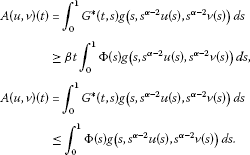Therefore, $A\left(u,v\right)\left(t\right)\ge \beta \parallel A\left(u,v\right)\parallel t$. Combining with (2.16), we have $A:Q×Q\to Q$. □

Remark 2.3 By (${H}_{2}$) and (2.15), A is a mixed monotone operator.

## 3 Main results

Theorem 3.1 Suppose that (${H}_{1}$)-(${H}_{3}$) hold. Then the BVP (1.1) has a unique positive solution.

Proof For any $r\in \left(0,1\right)$, by Remark 2.2, we have

$A\left(\frac{u}{r},rv\right)\le {r}^{-\mu }A\left(u,v\right),\phantom{\rule{1em}{0ex}}\mathrm{\forall }u,v\in Q.$

For any $w\in Q$, noticing $A\left(w,w\right)\in Q$, we can choose ${r}_{0}\in \left(0,1\right)$ small enough such that

${r}_{0}^{1-\mu }w\le A\left(w,w\right)\le {r}_{0}^{-\left(1-\mu \right)}w.$
(3.1)

Set

${u}_{0}={r}_{0}w,\phantom{\rule{2em}{0ex}}{v}_{0}={r}_{0}^{-1}w.$
(3.2)

Clearly,

${u}_{0},{v}_{0}\in Q,\phantom{\rule{2em}{0ex}}{u}_{0}\le {v}_{0}.$

Let

${u}_{n}=A\left({u}_{n-1},{v}_{n-1}\right),\phantom{\rule{2em}{0ex}}{v}_{n}=A\left({v}_{n-1},{u}_{n-1}\right),\phantom{\rule{1em}{0ex}}n=1,2,\dots .$
(3.3)

It is easy to see that

${u}_{0}\le {u}_{1}\le \cdots \le {u}_{n}\le \cdots \le {v}_{n}\le \cdots \le {v}_{1}\le {v}_{0}.$
(3.4)

Noticing

$\begin{array}{c}{u}_{1}=A\left({r}_{0}w,{r}_{0}^{-1}w\right)\ge {r}_{0}^{\mu }A\left(w,w\right),\hfill \\ {v}_{1}=A\left({r}_{0}^{-1}w,{r}_{0}w\right)\le {r}_{0}^{-\mu }A\left(w,w\right),\hfill \end{array}$

therefore,

${u}_{1}\ge {r}_{0}^{2\mu }{v}_{1}.$

Suppose that ${u}_{n}\ge {r}_{0}^{2{\mu }^{n}}{v}_{n}$, then ${v}_{n}\le {r}_{0}^{-2{\mu }^{n}}{u}_{n}$, and

${u}_{n+1}=A\left({u}_{n},{v}_{n}\right)\ge A\left({r}_{0}^{2{\mu }^{n}}{v}_{n},{r}_{0}^{-2{\mu }^{n}}{u}_{n}\right)\ge {r}_{0}^{2{\mu }^{n+1}}A\left({v}_{n},{u}_{n}\right).$

By induction, we can get

${u}_{n}\ge {r}_{0}^{2{\mu }^{n}}{v}_{n},\phantom{\rule{1em}{0ex}}n=1,2,\dots .$
(3.5)

By (3.4), (3.5), we have

$0\le {u}_{n+m}-{u}_{n}\le {v}_{n}-{u}_{n}\le \left(1-{r}_{0}^{2{\mu }^{n}}\right){v}_{n}\le \left(1-{r}_{0}^{2{\mu }^{n}}\right){v}_{0},$

which implies $\left\{{u}_{n}\right\}$ is a Cauchy sequence. Similarly, $\left\{{v}_{n}\right\}$ is a Cauchy sequence. Noticing (3.4), there exist ${u}^{\ast },{v}^{\ast }\in Q$, such that $\left\{{u}_{n}\right\}$ converges to ${u}^{\ast }$ and $\left\{{v}_{n}\right\}$ converges to ${v}^{\ast }$. Moreover,

${u}_{n}\le {u}^{\ast }\le {v}^{\ast }\le {v}_{n},\phantom{\rule{1em}{0ex}}n=1,2,\dots .$
(3.6)

(3.5) and (3.6) imply that

$\parallel {v}^{\ast }-{u}^{\ast }\parallel \le \parallel {v}_{n}-{u}_{n}\parallel \le \left(1-{r}_{0}^{{\mu }^{n}}\right)\parallel {v}_{0}\parallel ,\phantom{\rule{1em}{0ex}}n=1,2,\dots .$
(3.7)

This implies that ${u}^{\ast }={v}^{\ast }$.

By the mixed monotone property of A and (3.6), we have

$A\left({u}^{\ast },{v}^{\ast }\right)\ge A\left({u}_{n},{v}_{n}\right)={u}_{n+1},\phantom{\rule{2em}{0ex}}A\left({v}^{\ast },{u}^{\ast }\right)\le A\left({v}_{n},{u}_{n}\right)={v}_{n+1},\phantom{\rule{1em}{0ex}}n=1,2,\dots .$

Let $n\to +\mathrm{\infty }$, we get

${u}^{\ast }\le A\left({u}^{\ast },{v}^{\ast }\right)=A\left({v}^{\ast },{u}^{\ast }\right)\le {v}^{\ast }.$

Since ${u}^{\ast }={v}^{\ast }$, we have ${u}^{\ast }$ is a positive fixed point of A.

In the following, we will prove the positive fixed point of A is unique.

Suppose $u\ne {u}^{\ast }$ is a positive fixed point of A. By Lemma 2.6, we can get $u\in Q$. Let

${r}_{1}=sup\left\{r\in \left(0,1\right):r{u}^{\ast }\le u\le {r}^{-1}{u}^{\ast }\right\}.$

Then $0<{r}_{1}<1$, and ${r}_{1}{u}^{\ast }\le u\le {r}_{1}^{-1}{u}^{\ast }$. Therefore

$\begin{array}{c}u=A\left(u,u\right)\ge A\left({r}_{1}{u}^{\ast },{r}_{1}^{-1}{u}^{\ast }\right)\ge {r}_{1}^{\mu }A\left({u}^{\ast },{u}^{\ast }\right)={r}_{1}^{\mu }{u}^{\ast },\hfill \\ u=A\left(u,u\right)\le A\left({r}_{1}^{-1}{u}^{\ast },{r}_{1}{u}^{\ast }\right)\le {r}_{1}^{-\mu }A\left({u}^{\ast },{u}^{\ast }\right)={r}_{1}^{-\mu }{u}^{\ast }.\hfill \end{array}$

Thus, ${r}_{1}^{\mu }{u}^{\ast }\le u\le {r}_{1}^{-\mu }{u}^{\ast }$, which contradicts the definition of ${r}_{1}$. Consequently, the positive fixed point of A is unique.

It is clear that $y\left(t\right)={t}^{\alpha -2}{u}^{\ast }\left(t\right)$ satisfies

$y\left(t\right)={\int }_{0}^{1}G\left(t,s\right)g\left(s,y\left(s\right),y\left(s\right)\right)\phantom{\rule{0.2em}{0ex}}ds={\int }_{0}^{1}G\left(t,s\right)f\left(s,y\left(s\right)\right)\phantom{\rule{0.2em}{0ex}}ds,\phantom{\rule{1em}{0ex}}t\in \left(0,1\right].$

On the other hand, since ${u}^{\ast }\in Q$, we have $\beta \parallel {u}^{\ast }\parallel t\le {u}^{\ast }\left(t\right)\le {l}_{{u}^{\ast }}t$. Then, $\beta \parallel {u}^{\ast }\parallel {t}^{\alpha -1}\le y\left(t\right)\le {l}_{{u}^{\ast }}{t}^{\alpha -1}$. By Lemma 2.5 and (${H}_{2}$), (${H}_{3}$), we can get $g\left(t,y\left(t\right),y\left(t\right)\right)\in L\left(0,1\right)$. Moreover,

$\begin{array}{rcl}\underset{t\to 0}{lim}y\left(t\right)& =& \underset{t\to 0}{lim}{\int }_{0}^{1}G\left(t,s\right)g\left(s,y\left(s\right),y\left(s\right)\right)\phantom{\rule{0.2em}{0ex}}ds\\ \le & {t}^{\alpha -1}{\int }_{0}^{1}\left(\frac{1}{\mathrm{\Gamma }\left(\alpha \right)}+q\left(s\right)\right)g\left(s,y\left(s\right),y\left(s\right)\right)\phantom{\rule{0.2em}{0ex}}ds\\ =& 0.\end{array}$

Lemma 2.3 implies $y\left(t\right)={t}^{\alpha -2}{u}^{\ast }\left(t\right)$ is a positive solution of (1.1).

On the other hand, if $y\left(t\right)$ is a positive solution of (1.1), then

$y\left(t\right)={\int }_{0}^{1}G\left(t,s\right)g\left(s,y\left(s\right),y\left(s\right)\right)\phantom{\rule{0.2em}{0ex}}ds.$

By Lemma 2.5, we have there exists ${l}_{1},{l}_{2}>0$ such that

${l}_{2}{t}^{\alpha -1}\le y\left(t\right)\le {l}_{1}{t}^{\alpha -1}.$

Set $u\left(t\right)={t}^{2-\alpha }y\left(t\right)$, we have

${l}_{2}t\le u\left(t\right)\le {l}_{1}t,$

and

$u\left(t\right)={\int }_{0}^{1}{G}^{\ast }\left(t,s\right)g\left(s,{s}^{\alpha -2}u\left(s\right),{s}^{\alpha -2}v\left(s\right)\right)\phantom{\rule{0.2em}{0ex}}ds,$

which implies u is a positive fixed point of A.

Then $y\left(t\right)={t}^{\alpha -2}{u}^{\ast }\left(t\right)$ is the unique positive solution of the BVP (1.1). □

Remark 3.1 The unique positive solution y of (1.1) can be approximated by the iterative schemes: for any $w\in Q$, let ${u}_{0}$, ${v}_{0}$ be defined as (3.2) and ${u}_{n}=A\left({u}_{n-1},{v}_{n-1}\right)$, ${v}_{n}=A\left({v}_{n-1},{u}_{n-1}\right)$, $n=1,2,\dots$ , then ${t}^{\alpha -2}{u}_{n}\to y$.

Example 3.1 (A 4-point BVP with coefficients of both signs)

Consider the following problem:

$\left\{\begin{array}{c}{D}_{0+}^{\frac{7}{4}}u\left(t\right)+f\left(t,u\left(t\right)\right)=0,\phantom{\rule{1em}{0ex}}t\in \left(0,1\right),\hfill \\ u\left(0\right)=0,\phantom{\rule{2em}{0ex}}{D}_{0+}^{\frac{1}{4}}u\left(1\right)={D}_{0+}^{\frac{1}{4}}u\left(\frac{1}{4}\right)-\frac{1}{2}{D}_{0+}^{\frac{1}{4}}u\left(\frac{4}{9}\right)\hfill \end{array}$
(3.8)

with

$f\left(t,x\right)=-{x}^{\frac{1}{2}}lnt-{x}^{-\frac{1}{3}}ln\left(1-t\right)+{x}^{-\frac{1}{3}}ln\left(1+{x}^{\frac{1}{2}}\right).$

Then

${G}_{0}\left(t,s\right)=\frac{1}{\mathrm{\Gamma }\left(\frac{7}{4}\right)}\left\{\begin{array}{cc}{t}^{\frac{3}{4}}{\left(1-s\right)}^{\frac{1}{2}},\hfill & 0\le t\le s\le 1,\hfill \\ {t}^{\frac{3}{4}}{\left(1-s\right)}^{\frac{1}{2}}-{\left(t-s\right)}^{\frac{3}{4}},\hfill & 0\le s\le t\le 1\hfill \end{array}$

and

$p\left(s\right)=\left\{\begin{array}{cc}1-{\left(\frac{\frac{1}{4}-s}{1-s}\right)}^{\frac{1}{2}}-\frac{1}{2}{\left(\frac{\frac{4}{9}-s}{1-s}\right)}^{\frac{1}{2}},\hfill & 0\le s\le \frac{1}{4},\hfill \\ 1-\frac{1}{2}{\left(\frac{\frac{4}{9}-s}{1-s}\right)}^{\frac{1}{2}},\hfill & \frac{1}{4}

By direct calculations, we have $p\left(0\right)=\frac{5}{6}$ and $q\left(s\right)\ge 0$, which implies (${H}_{1}$) holds.

Let

$g\left(t,x,y\right)=-{x}^{\frac{1}{2}}lnt-{y}^{-\frac{1}{3}}ln\left(1-t\right)+{y}^{-\frac{1}{3}}ln\left(1+{x}^{\frac{1}{2}}\right).$

Obviously, $g\in C\left(\left(0,1\right)×\left[0,+\mathrm{\infty }\right)×\left(0,+\mathrm{\infty }\right)\to \left[0,+\mathrm{\infty }\right)\right)$, $g\left(t,x,y\right)$ is nondecreasing on x and nonincreasing on y. It is easy to see that

$ln\left(1+rx\right)\ge rln\left(1+x\right),\phantom{\rule{1em}{0ex}}\mathrm{\forall }x\ge 0,r\in \left(0,1\right).$
(3.9)

Then

$g\left(t,rx,\frac{y}{r}\right)\ge {r}^{\frac{5}{6}}g\left(t,x,y\right),\phantom{\rule{1em}{0ex}}\mathrm{\forall }x,y>0,r\in \left(0,1\right).$

Therefore (${H}_{2}$) holds. It is easy to get that (${H}_{3}$) holds. Therefore, the assumptions of Theorem 3.1 are satisfied. Thus Theorem 3.1 ensures that the BVP (3.8) has a unique positive solution.

## References

1. Samko SG, Kilbas AA, Marichev OI: Fractional Integral and Derivatives (Theory and Applications). Gordon & Breach, Switzerland; 1993.

2. Podlubny I Mathematics in Science and Engineering 198. In Fractional Differential Equations. Academic Press, New York; 1999.

3. Kilbas AA, Srivastava HM, Trujillo JJ: Theory and Applications of Fractional Differential Equations. Elsevier, Amsterdam; 2006.

4. Agrawal OP: Formulation of Euler-Lagrange equations for fractional variational problems. J. Math. Anal. Appl. 2002, 272: 368-379. 10.1016/S0022-247X(02)00180-4

5. Lakshmikantham V, Vatsala AS: General uniqueness and monotone iterative technique for fractional differential equations. Appl. Math. Lett. 2008, 21: 828-834. 10.1016/j.aml.2007.09.006

6. Kosmatov N: Integral equations and initial value problems for nonlinear differential equations of fractional order. Nonlinear Anal. 2009, 70: 2521-2529. 10.1016/j.na.2008.03.037

7. Agarwal RP, O’Regan D, Stanek S: Positive solutions for Dirichlet problem of singular nonlinear fractional differential equations. J. Math. Anal. Appl. 2010, 371: 57-68. 10.1016/j.jmaa.2010.04.034

8. Ahmad B, Alsaedi A: Existence of solutions for anti-periodic boundary value problems of nonlinear impulsive functional integro-differential equations of mixed type. Nonlinear Anal. 2009, 3: 501-509.

9. Liang S, Zhang J: Positive solutions for boundary value problems of fractional differential equation. Nonlinear Anal. 2009, 71: 5545-5550. 10.1016/j.na.2009.04.045

10. Jiang D, Yuan C: The positive properties of the Green function for Dirichlet-type boundary value problems of nonlinear fractional differential equations and its application. Nonlinear Anal. 2010, 72: 710-719. 10.1016/j.na.2009.07.012

11. Goodrich CS: Existence of positive solution to a class of fractional differential equations. Appl. Math. Lett. 2010, 23: 1050-1055. 10.1016/j.aml.2010.04.035

12. Arara A, Benchohra M, Hamidi N, Nieto JJ: Fractional order differential equations on an unbounded domain. Nonlinear Anal. 2010, 72: 580-586. 10.1016/j.na.2009.06.106

13. Salem HAH: On the fractional order m -point boundary value problem in reflexive Banach spaces and weak topologies. Comput. Math. Appl. 2009, 224: 565-572. 10.1016/j.cam.2008.05.033

14. Li CF, Luo XN, Zhou Y: Existence of positive solutions of the boundary value problem for nonlinear fractional differential equations. Comput. Math. Appl. 2010, 59: 1363-1375. 10.1016/j.camwa.2009.06.029

15. Benchohraa M, Hamania S, Ntouyas SK: Boundary value problems for differential equations with fractional order and nonlocal conditions. Nonlinear Anal. 2009, 71: 2391-2396. 10.1016/j.na.2009.01.073

16. El-Shahed M, Nieto JJ: Nontrivial solutions for a nonlinear multi-point boundary value problem of fractional order. Comput. Math. Appl. 2010, 59: 3438-3443. 10.1016/j.camwa.2010.03.031

17. Bai Z: On positive solutions of a nonlocal fractional boundary value problem. Nonlinear Anal. 2010, 72: 916-924. 10.1016/j.na.2009.07.033

18. Rehman M, Khan RA: Existence and uniqueness of solution for multi-point boundary value problems for fractional differential equations. Appl. Math. Lett. 2010, 23: 1038-1044. 10.1016/j.aml.2010.04.033

19. Allison J, Kosmatov N: Multi-point boundary value problems of fractional order. Commun. Appl. Anal. 2008, 12(4):451-458.

20. Zhang S: Positive solution to singular boundary value problem for nonlinear fractional differential equation. Comput. Math. Appl. 2010, 59: 1300-1309. 10.1016/j.camwa.2009.06.034

21. Yang L, Chen H: Unique positive solutions for fractional differential equation boundary value problems. Appl. Math. Lett. 2010, 23: 1095-1098. 10.1016/j.aml.2010.04.042

22. Chang Y, Kavitha V, Mallika Arjunan M: Existence and uniqueness of mild solutions to a semilinear integrodifferential equation of fractional order. Nonlinear Anal. 2009, 71: 5551-5559. 10.1016/j.na.2009.04.058

23. Shua X, Lai Y, Chen Y: The existence of mild solutions for impulsive fractional partial differential equations. Nonlinear Anal. 2011, 74: 2003-2011. 10.1016/j.na.2010.11.007

## Acknowledgements

The authors are grateful to the anonymous referee for his/her valuable suggestions. The first and second authors were supported financially by the National Natural Science Foundation of China (11071141, 11126231) and Project of Shandong Province Higher Educational Science and Technology Program (J11LA06). The third author was supported financially by the Australia Research Council through an ARC Discovery Project Grant.

## Author information

Authors

### Corresponding author

Correspondence to Yongqing Wang.

### Competing interests

The authors declare that they have no competing interests.

### Authors’ contributions

The authors declare that the study was realized in collaboration with the same responsibility. All authors read and approved the final manuscript.

## Rights and permissions

Reprints and Permissions

Wang, Y., Liu, L. & Wu, Y. Existence and uniqueness of positive solution to singular fractional differential equations. Bound Value Probl 2012, 81 (2012). https://doi.org/10.1186/1687-2770-2012-81

• Accepted:

• Published:

• DOI: https://doi.org/10.1186/1687-2770-2012-81

### Keywords

• fractional differential equation
• positive solution
• iterative scheme
• singular boundary value problem